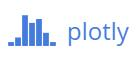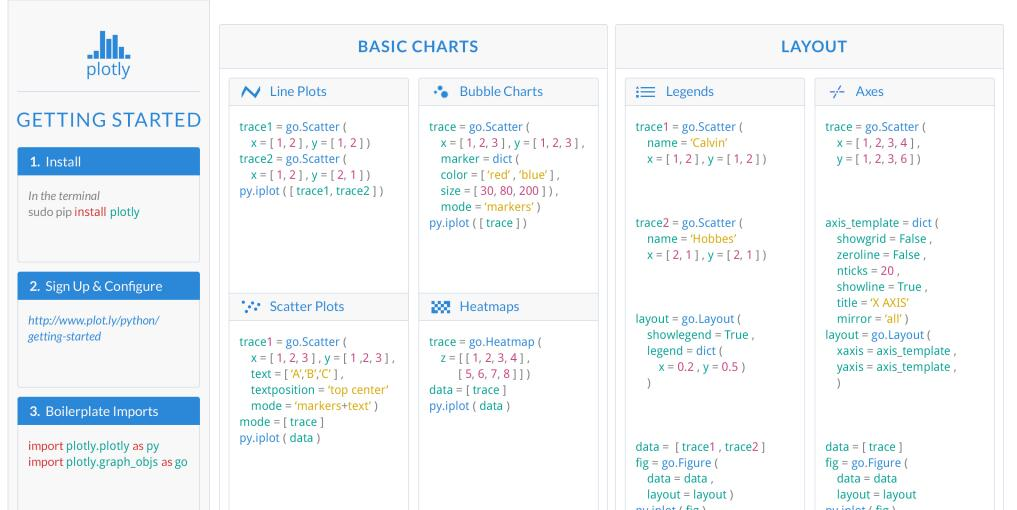# Python数据可视化：顶级绘图库plotly有史以来最牛逼的绘图工具，没有之一
plotly是现代平台的敏捷商业智能和数据科学库，它作为一款开源的绘图库，可以应用于Python、R、MATLAB、Excel、JavaScript和jupyter等多种语言，主要使用的js进行图形绘制，实现过程中主要就是调用plotly的函数接口，底层实现完全被隐藏，便于初学者的掌握。

下面主要从Python的角度来分析plotly的绘图原理及方法：

###安装plotly：
使用pip来安装plotly库，如果机器上没有pip，需要先进行pip的安装，这里主要介绍plotly的安装。

$pip install plotly or$ sudo pip install plotly
or update


###输出方式：

将你的可视化图像保存到网站上，便于共享和保存。

import plotly.plotly as py
import plotly.graph_objs as go

py.sign_in('DemoAccount', '2qdyfjyr7o') # 注意：这里是plotly网站的用户名和密码

trace = go.Bar(x=[2, 4, 6], y= [10, 12, 15])
data = [trace]
layout = go.Layout(title='A Simple Plot', width=800, height=640)
fig = go.Figure(data=data, layout=layout)

py.image.save_as(fig, filename='a-simple-plot.png')

from IPython.display import Image
Image('a-simple-plot.png')


直接在本地生成可视化图像，便于使用。

# -*- coding:utf-8 -*-

import plotly.plotly
import plotly.graph_objs as go

trace = go.Box(
x=[1, 2, 3, 4, 5, 6, 7]
)
data = [trace]
plotly.offline.plot(data)  # 离线方式使用：offline


###plotly绘图：

• 基本图表：20种
• 统计和海运方式图：12种
• 科学图表：21种
• 财务图表：2种
• 地图：8种
• 3D图表：19种
• 报告生成：4种
• 连接数据库：7种
• 拟合工具：3种
• 流动图表：4种
• JavaScript添加自定义控件：13种©️2019 CSDN 皮肤主题: 技术黑板 设计师: CSDN官方博客